# Coulomb's law is similar to Newton's law of gravitation in several ways. Which one of the...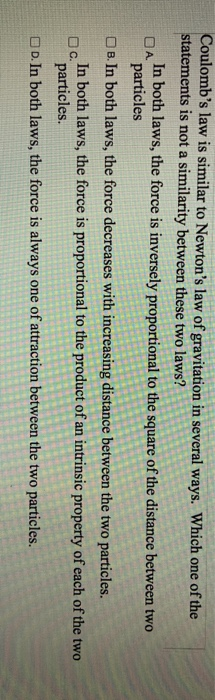Coulomb's law is similar to Newton's law of gravitation in several ways. Which one of the statements is not a similarity between these two laws? In both laws, the force is inversely proportional to the square of the distance between two ОА. particles B. In both laws, the force decreases with increasing distance between the two particles. In both laws, the force is proportional to the product of an intrinsic property of each of the two particles. D. In both laws, the force is always one of attraction between the two particles. C.Thanks! Please rate it up

#### Earn Coin

Coins can be redeemed for fabulous gifts.

Similar Homework Help Questions
• ### Two 639-kg masses are separated by a distance of 0.15 m.  Using Newton's Law of Universal Gravitation,...

Two 639-kg masses are separated by a distance of 0.15 m.  Using Newton's Law of Universal Gravitation, find the gravitational force of attraction between these two masses.

• ### Given Newton's law of universal gravitation where F is the force between two masses objects, m1...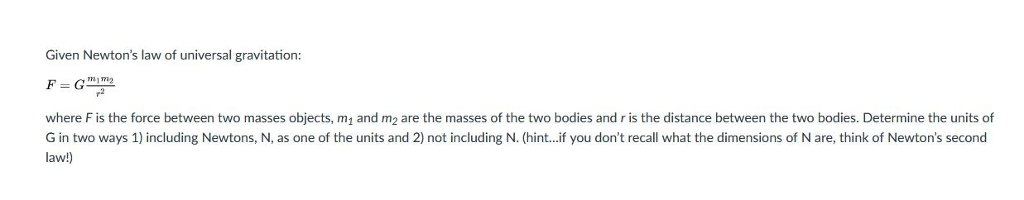Given Newton's law of universal gravitation where F is the force between two masses objects, m1 and m2 are the masses of the two bodies and r is the distance between the two bodies. Determine the units of G in two ways 1) including Newtons, N, as one of the units and 2) not including N. (hint...if you don't recall what the dimensions of N are, think of Newton's second law!

• ### 3. (6 pts) Newton's law of gravity and Coulomb's law are both inverse-square laws. Consequently, there...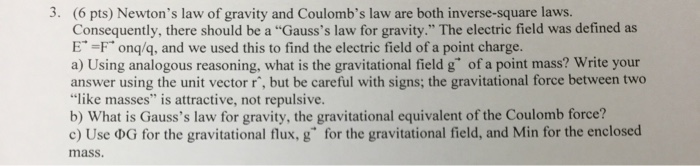3. (6 pts) Newton's law of gravity and Coulomb's law are both inverse-square laws. Consequently, there should be a "Gauss's law for gravity." The electric field was defined as E" =F" onq/q, and we used this to find the electric field of a point charge. a) Using analogous reasoning, what is the gravitational field g" of a point mass? Write your answer using the unit vector r', but be careful with signs; the gravitational force between two "like masses" is...

• ### I need help with question 41. (e) magnetic intensity 38. Acconding to Coulomb's Law, the fonce of interaction between 2 charged paerticles is innversely proportional to the square of their sep...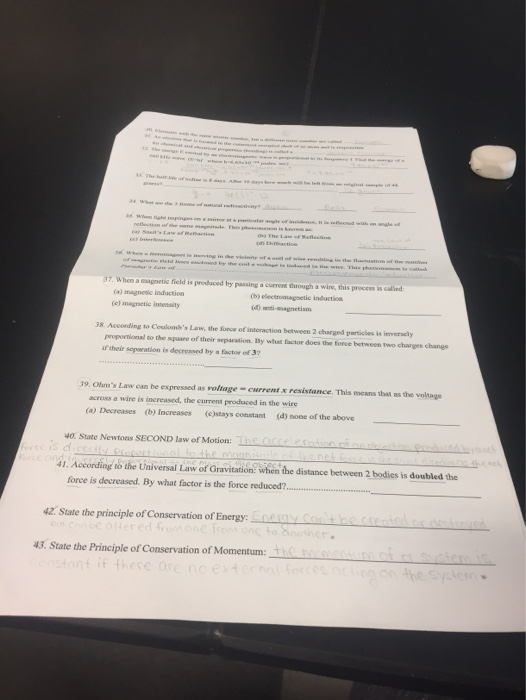I need help with question 41. (e) magnetic intensity 38. Acconding to Coulomb's Law, the fonce of interaction between 2 charged paerticles is innversely proportional to the square of their separation IBty what factor does the torce between two charges chanes i their sepwration is decreased by a factor of 3 current x resistance. This means that as the voltage across a wire is increased, the current produced in the wire (b) Increases (d) none of the above (a) Decreases...

• ### Learning Goal: To understand Newton's law of gravitation and the distinction between inertial and gravitational masses....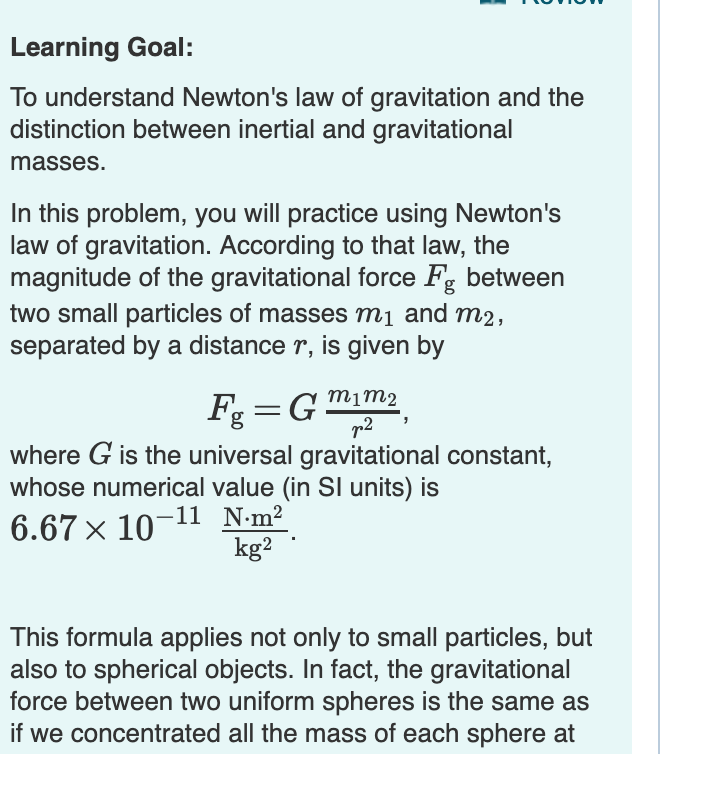Learning Goal: To understand Newton's law of gravitation and the distinction between inertial and gravitational masses. In this problem, you will practice using Newton's law of gravitation. According to that law, the magnitude of the gravitational force Fg between two small particles of masses m1 and m2 separated by a distance r, is given by m1m2 T2 where G is the universal gravitational constant, whose numerical value (in SI units) is 6.67 x 10-11 Nm2 kg2 This formula applies not...

• ### Use Newton's law of universal Gravitation to estimate force exerted by one object on another: F...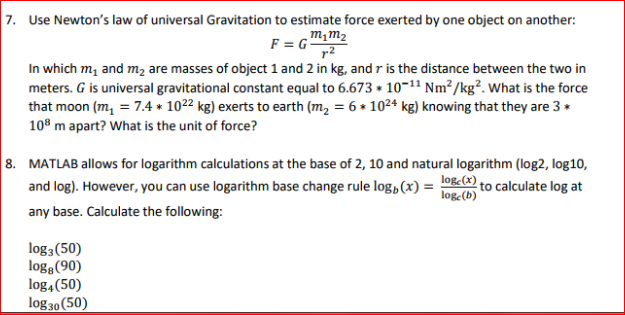Use Newton's law of universal Gravitation to estimate force exerted by one object on another: F = G m_1 m_2/r^2 In which m_1 and m_2 are masses of object 1 and 2 in kg, and r is the distance between the two in meters. G is universal gravitational constant equal to 6.673 * 10^-11 Nm ^2/kg^2. What is the force that moon (m_l = 7.4 * 10^22 kg) exerts to earth (m_2 = 6 * 10^24 kg) knowing that they...

• ### Newton's Law of Gravitation states that two bodies with masses my and m2 attract each other...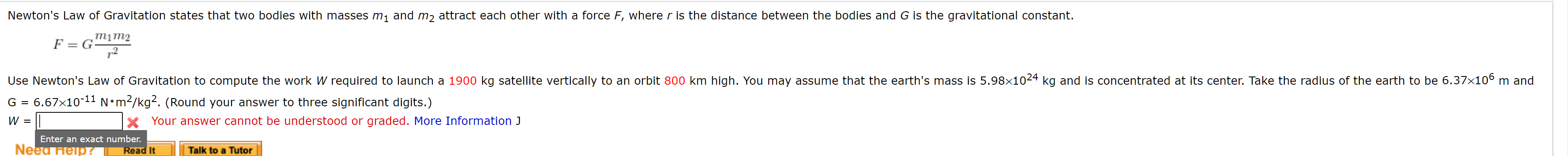Newton's Law of Gravitation states that two bodies with masses my and m2 attract each other with a force F, where r is the distance between the bodies and G is the gravitational constant. mim2 F=G 72 Use Newton's Law of Gravitation to compute the work W required to launch a 1900 kg satellite vertically to an orbit 800 km high. You may assume that the earth's mass is 5.98x1024 kg and is concentrated at its center. Take the radius...

• ### out of 1.00 Questions Newton's law for universal gravitation can be written as: F = mm...out of 1.00 Questions Newton's law for universal gravitation can be written as: F = mm where in the universal gravitational Constantin Nm .m, and are the masses in kg and is the distance between the centre of mass of mand me in m. The force on mass mis towards mass my. Let stand for the distance from the centre of mass of a planet with mass M. A satellite with mass mis moved along a straight line from x...

• ### Laboratory Unversal Gravitational Law please answer all 3 cases. thank you Laboratory universal gravitational law Answer...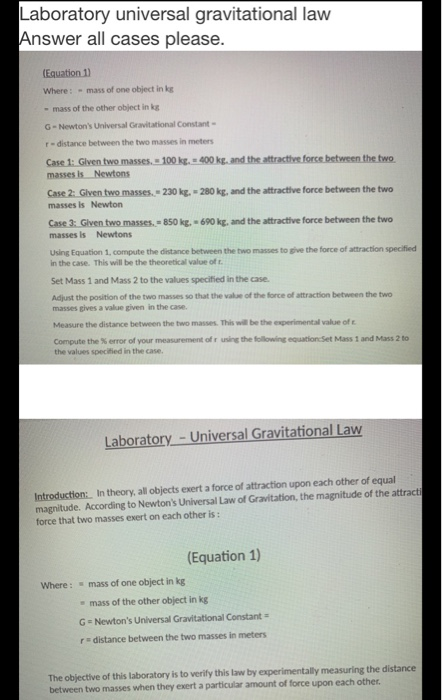Laboratory Unversal Gravitational Law please answer all 3 cases. thank you Laboratory universal gravitational law Answer all cases please. (Equation 11 Where: - mass of one object in ks - mass of the other object in kg G-Newton's Universal Gravitational Constant r - distance between the two masses in meters Case 1: Glven two masses. - 100 kg = 400 kg, and the attractive force between the two masses is Newtons Case 2: Glven two masses... 230 kg. - 280...

• ### 1. Which characteristic of Coulomb's law is described or identified by Newton's third law of (1...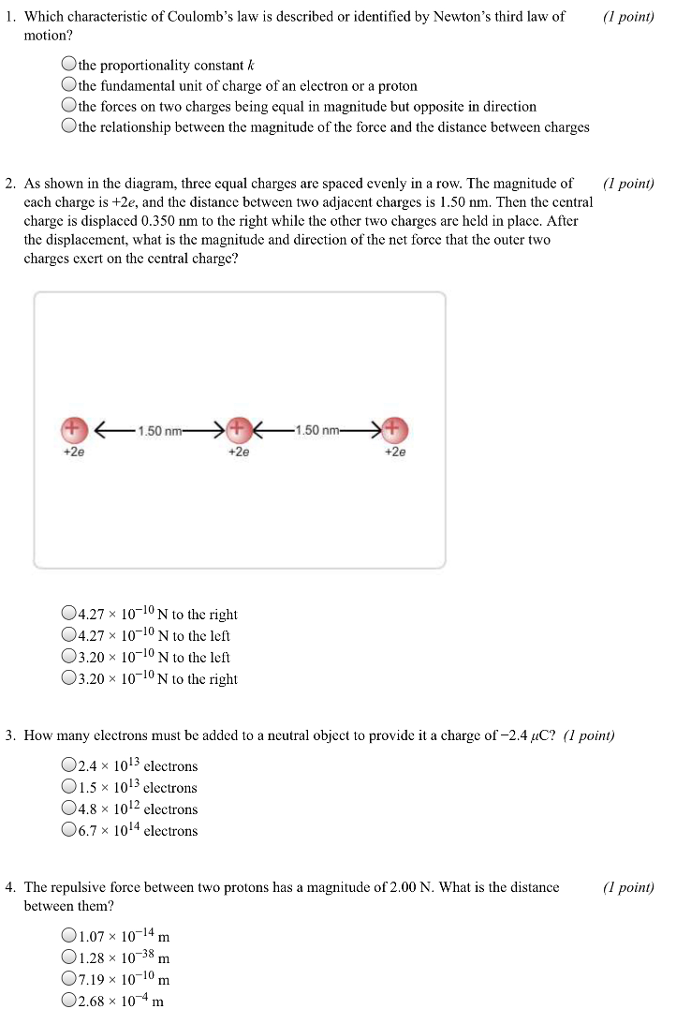1. Which characteristic of Coulomb's law is described or identified by Newton's third law of (1 point) motion? Othe proportionality constant k the fundamental unit of charge of an electron or a proton Othe forces on two charges being equal in magnitude but opposite in direction Othe relationship between the magnitude of the force and the distance between charges 2. As shown in the diagram, three equal charges are spaced evenly in a row. The magnitude of point) cach charge...

Free Homework App